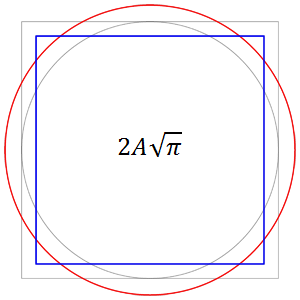$\pi$ is not transcendental? Say what?

Logic Level 3Which step did I commit a fallacy?

Step 1:

With my calculator, I get $\sin \left (2017 \sqrt{2} \right) = -1 = \sin \left (-\frac {\pi}{2} \right )$

Step 2:

Because the $f(x) =\sin(x)$ has period $2\pi$,

$2017 \sqrt{2} = -\frac {\pi}{2} + 2\pi n$, for a certain integer $n$

Step 3:

Rearrange the equation and set $\pi$ as the subject

$\LARGE \pi = \frac {2017 \cdot 2^{6/5} }{4n - 1}$

This means $\pi$ is not a transcendental number but an algebraic number.

×